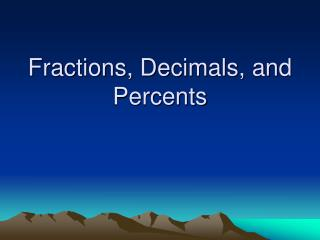DownloadDownload PresentationFractions, Decimals, and Percents

# Fractions, Decimals, and Percents

Télécharger la présentation## Fractions, Decimals, and Percents

- - - - - - - - - - - - - - - - - - - - - - - - - - - E N D - - - - - - - - - - - - - - - - - - - - - - - - - - -
##### Presentation Transcript

1. Fractions, Decimals, and Percents

2. Write these percents as decimals… • 58% = 0.58 read “58 hundredths” • 3% = 0.03 read “3 hundredths” • 12.5% = 0.125 read “125 thousandths”

3. Write these percents as reduced fractions… • 71% = 71/100 • 12% = 12/100 = 6/50 = 3/25 • 150% = 150/100 = 3/2 = 1 and 1/2

4. Write these decimals as percents… • 0.05 = 5% • 1.33 = 133% • “Twenty five thousandths” = 2.5%

5. Write these decimals as reduced fractions … • Hint: It can help if you say the fraction. • 0.25 = 25/100 = 1/4 • 2.24 = 2 and 24/100 = 2 and 12/50 = 2 and 6/25 • 0.005 = 5/1000 = 1/200

6. Fraction to % when the denominator is a factor of 100 … • 17/25 = ____ % 17/25 x 4/4 = 68/100 = 68% • 3/20 = ____ % 3/20 x 5/5 = 15/100 = 15% • 1/5 = 1/5 x 20/20 = 20/100 = 20%

7. Fraction to % when the denominator is not a factor of 100 • 1/8 = ____ % 1/8 = 0.125 = 12.5% • 1/6 = ____ % 1/6 = 0.1666 = 16.7% • 4/9 = 4/9 = 0.444 = 44.4%

8. Quick Hitters • 1/3 = ___ % 1/100 = ___ % ¾ = ___% 33.3% 1% 75% • 1/2 = ___ % 2/5 = ___ % 3/5 = ___% 50% 40% 60% • 2/3 = ___ % 1/5 = ___ % 4/5 = ___% 66.6% 20% 80%

9. Good Job! • HW P. 785 #1-45 Odds • If you forget how to solve a certain type, look for examples on pages 784 and 785!